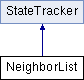ParM  parm A molecular dynamics library
NeighborList Class Reference

Maintains a Verlet list of "neighbors": molecules within a 'skin radius' of each other. More...

#include <trackers.hpp>

Inheritance diagram for NeighborList:## Public Member Functions

NeighborList (sptr< Box > box, sptr< AtomVec > atoms, const flt skin)

void update (Box &newbox)
Calls update_list(false); exists as a requirement of StateTracker. More...

bool update_list (bool force=true)
Update the neighbor list based on current positions. More...

AtomVecvec ()

uint which ()

uint numpairs ()

void ignore (AtomID a, AtomID b)

void add (AtomID a, flt diameter)

uint ignore_size () const
Number of pairs currently being ignored. More...

uint size () const
Number of atoms in list. More...

vector< IDPair >::iterator begin ()

vector< IDPair >::iterator end ()

IDPair get (uint i)

~NeighborList ()Public Member Functions inherited from StateTracker
virtual ~StateTracker ()

## Protected Attributes

sptr< Boxbox

flt skin

SubGroup atoms

vector< fltdiameters

vector< IDPaircurpairs

PairList ignorepairs

vector< Veclastlocs

uint updatenum

bool ignorechanged

## Detailed Description

Maintains a Verlet list of "neighbors": molecules within a 'skin radius' of each other.

When the list is updated (update_list(true)), molecules are counted as neighbors if the distance between them is $$d_{ij} < r_i + r_j + R$$, where $$r_i$$ and $$r_j$$ are the radii, and $$R$$ is the "skin radius". On "normal" updates (update(box) or update_list(false), All atoms fulfilling the condition $$d_{ij} < r_i + r_j$$ are guaranteed to be in the list, but there may be some false positives.

update_list(false) should be called frequently (i.e. every timestep); it checks ( $$O(N)$$) if any two molecules might conceivably overlap by more than a critical distance, and if so, it updates all the neighbor lists.

The NeighborList has an inherent tradeoff, set by the skin radius: If the skin is large, then full updates (which are slow) will be needed less often, but more particles will be considered "neighbors" even when they are not within $$d_{ij} < r_i + r_j$$, i.e. there will be more false positives.

## Constructor & Destructor Documentation

 NeighborList::NeighborList ( sptr< Box > box, sptr< AtomVec > atoms, const flt skin )
 NeighborList::~NeighborList ( )
inline

## Member Function Documentation

 void NeighborList::add ( AtomID a, flt diameter )
inline
 vector::iterator NeighborList::begin ( )
inline
 vector::iterator NeighborList::end ( )
inline
 IDPair NeighborList::get ( uint i )
inline
 void NeighborList::ignore ( AtomID a, AtomID b )
inline
 uint NeighborList::ignore_size ( ) const
inline

Number of pairs currently being ignored.

 uint NeighborList::numpairs ( )
inline
 uint NeighborList::size ( ) const
inline

Number of atoms in list.

 void NeighborList::update ( Box & newbox )
inlinevirtual

Calls update_list(false); exists as a requirement of StateTracker.

Implements StateTracker.

 bool NeighborList::update_list ( bool force = true )

Update the neighbor list based on current positions.

If force, the list is updated immediately $$O(N^2)$$; otherwise, NeighborList checks if an update is necessary $$O(N)$$, and updates the list if so.

 AtomVec& NeighborList::vec ( )
inline
 uint NeighborList::which ( )
inline

## Member Data Documentation

 SubGroup NeighborList::atoms
protected
 sptr NeighborList::box
protected
 vector NeighborList::curpairs
protected
 vector NeighborList::diameters
protected
 bool NeighborList::ignorechanged
protected
 PairList NeighborList::ignorepairs
protected
 vector NeighborList::lastlocs
protected
 flt NeighborList::skin
protected
 uint NeighborList::updatenum
protected

The documentation for this class was generated from the following files: